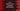# C++ program to check if a number is greater than another by using isgreater## C++ program to check if a number is greater than another by using isgreater:

In this post, we will learn how to check if a number is greater than another number or not in C++. We will use the isgreater function to check that.

isgreater is defined in cmath header. With this post, you will learn how to use isgreater with examples.

### Definition of isgreater:

isgreater method is defined as like below(in C++11):

``````bool isgreater (float x, float y);
bool isgreater (double x, double y);
bool isgreater (long double x, long double y);``````
• This method takes two parameters, i.e. the two numbers to compare.
• It returns one boolean value. It checks whether x is greater than y or not. i.e. if x>y, it returns true, else false.
• It has three overloaded methods in C++11 those take float, double, and long double values as the parameters.

### Example of isgreater:

Let’s try isgreater with an example:

``````#include <iostream>
#include <cmath>

int main ()
{
std::cout<<std::isgreater(5, 3)<<'\n';
std::cout<<std::isgreater(5.3, 33.1)<<'\n';
std::cout<<std::isgreater(5, 5)<<'\n';

return 0;
}``````

It will return true only for the first one.

``````1
0
0``````

### Check if a number is greater than another number by using isgreater:

Let’s use isgreater to check if a number is greater than another number or not and print one message based on its return value:

``````#include <iostream>
#include <cmath>

int main ()
{
double num1 = 33.43;
double num2 = 22.11;

if(std::isgreater(num1, num2)){
std::cout<<"num1 is greater than num2"<<'\n';
}else{
std::cout<<"num1 is smaller than num2"<<'\n';
}

return 0;
}``````

It is similar to the above program. The only difference is that we are using the result of isgreater in an if-else block and based on its return value we are printing a message.

It checks if num1 is greater than num2 or not.

If you run the above program, it will print:

``num1 is greater than num2``